Welcome

It is highly recommended that you have Javascript enabled; many features will not work unless you do. The recommended browsers are Firefox and Chrome; the board is also NOT mobile-friendly.

If you were referred by someone (ex. me, Jessica) please put their username in the referral box on the registration page. Ask them if you don't know their username.

If you are visiting for TESTING PURPOSES ONLY, this is the test account information:

Test

Select a forum to post in:

## Significant Figures

Science topics

Moderators: Jessica, Teachers

Looking for a topic?
View the Science Topics Directory to find topics quicker under different kinds of science.

### Significant Figures

Rules for counting significant figures

Zeros within a number are always significant. Both 4308 and 40.05 contain four significant figures.

Zeros that do nothing but set the decimal point are not significant. Thus, 470,000 has two significant figures.

Trailing zeros that aren't needed to hold the decimal point are significant. For example, 4.00 has three significant figures.

TRICK:

If a decimal is Present, start at the Pacific (LEFT) and keep crossing out the zeros until you reach the first non-zero number. Anything not crossed out is a significant figure
0.00502
--> cross out zeros until you reach the first non-zero number -- in this case, 5. There are 3 sig figs: 5, 0, and 2

If a decimal is Absent, start at the Atlantic (RIGHT) and keep crossing out the zeros until you reach the first non-zero number.
830,000
cross out zeros until you reach the first non-zero number....3. There are two sig figs: 8 and 3. <--

Addition and Subtraction with Significant Figures

When combining measurements with different degrees of accuracy and precision, the accuracy of the final answer can be no greater than the least accurate measurement. This principle can be translated into a simple rule for addition and subtraction: When measurements are added or subtracted, the answer can contain no more decimal places than the least accurate measurement.
150.0 g H[sub]2[/sub]O
+0.507 g salt
150.5 g solution

Multiplication and Division With Significant Figures

The same principle governs the use of significant figures in multiplication and division: the final result can be no more accurate than the least accurate measurement. In this case, however, we count the significant figures in each measurement, not the number of decimal places: When measurements are multiplied or divided, the answer can contain no more significant figures than the least accurate measurement.

Example: To illustrate this rule, let's calculate the cost of the copper in an old penny that is pure copper. Let's assume that the penny has a mass of 2.531 grams, that it is essentially pure copper, and that the price of copper is 67 cents per pound. We can start by from grams to pounds.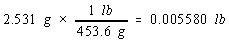We then use the price of a pound of copper to calculate the cost of the copper metal.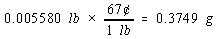There are four significant figures in both the mass of the penny (2.531) and the number of grams in a pound (453.6). But there are only two significant figures in the price of copper, so the final answer can only have two significant figures.

Rounding Off

When the answer to a calculation contains too many significant figures, it must be rounded off.

There are 10 digits that can occur in the last decimal place in a calculation. One way of rounding off involves underestimating the answer for five of these digits (0, 1, 2, 3, and 4) and overestimating the answer for the other five (5, 6, 7, 8, and 9). This approach to rounding off is summarized as follows.

If the digit is smaller than 5, drop this digit and leave the remaining number unchanged. Thus, 1.684 becomes 1.68.

If the digit is 5 or larger, drop this digit and add 1 to the preceding digit. Thus, 1.247 becomes 1.25.
• 0Jessica
Board Owner
Topic Author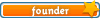Sapphire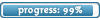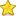Posts: 3,470
Topics: 1,244
Articles: 30
Joined: December 22nd, 2010, 8:04 pm